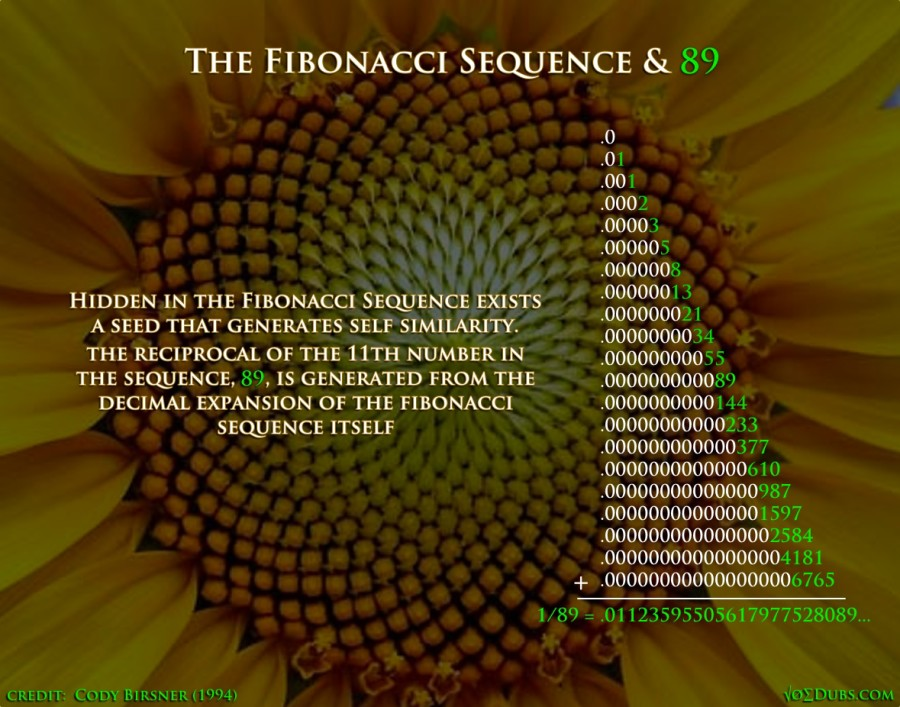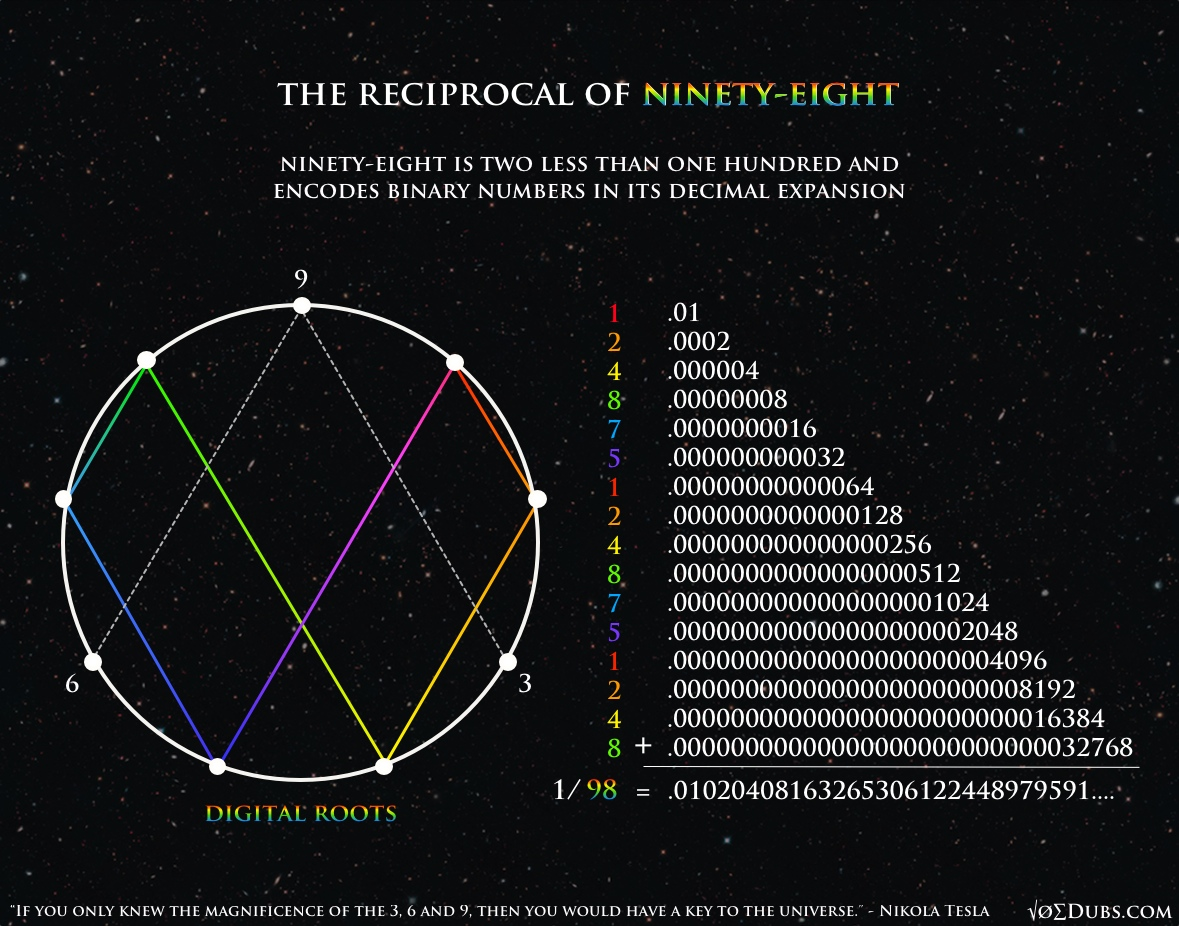# Fibonacci: The Fractions of Life

The Fibonacci Sequence is Nature’s favorite series of numbers.  They are found wherever there is life.  Closely associated with phi, this sequence actually generates this golden ratio when any number is divided by the number before it.

Phi = 1.618033988..

The Fibonacci Sequence: 0, 1, 1, 2, 3, 5, 8, 13, 21, 34, 55, 89, 144, 233, 377, 610, 1597, 2584, 4181, 6765, 10946, 17711, 28657, 46368, …

Phi and phee are generated from the Fibonacci sequence in this way.  This might be an indication that fractions are important, especially in relation to the Fibonacci numbers, since they create the golden ratio or the divine proportion.

The 11th number in the sequence is 89.  Zero is not a number but a placeholder for numeric expansion and contraction.  Interestingly 89 is 11 less than one hundred, but even more interesting is its reciprocal, which is 1/89.The decimal expansion of 1/89 is the Fibonacci series, added together in this manner.  Cody Birsner figured this out working on his Fibonacci term paper at the University of Oklahoma in 1994.   One divided by the eleventh number in the sequence creates the sequence itself through self-similar decimal expansion.

Let’s do 98If you add up decimals in this way ad infinitum, you’ll arrive at the reciprocal of 98 (1/98), which is .0102040….

The reciprocal of 49, another very auspicious number, generates the doubling pattern found in nature.  But lets not get off topic, Back to Fibonacci fractions…

The first twelve numbers in the Fibonacci sequence pair with the next twelve to create a male and female polarity with combined digital roots of 9. This pattern repeats to infinity. 89 is paired with 28,657.  (89 reduces to 8) and (28,657 reduces to 1)

This pattern of 24 repeats to infinity. The first twelve numbers pair with the second group of twelve to always reduce to nine.  Many thanks to Anthony Morris for this great infographic.

It makes sense that the Fibonacci sequence encodes the seed for self generation in its eleventh unit (89), as twelve marks completion in the cycle.  These golden numbers are holographic.  The whole can be made from just a piece.

The reciprocal of 28,657 also encodes the Fibonacci numbers.  This Fibonacci resonance is directly tied to 89 through their digital root pairing, so this is no surprise.  These reciprocal correlations are unique to these numbers.  In other words 1/144 or 1/233, etc,  doesn’t reveal anything interesting.

1 / 28,657 = .0000348954880133998673971455490805038908469134940852…

34 and 89 are the 9th and 11th Fibonacci numbers. Next is 54, one short of 55, and 88, one short of 89. Then comes 13.  It breaks down again until you hit 55 and then 08, which continues with 4 more Fibonacci numbers in a row (08,05,03,89,08)  Now this could always be coincidence, but I think you will see that with numbers, there is no such thing.

“Nothing in nature is by chance… Something appears to be chance only because of our lack of knowledge. ”
~ Baruch de Spinoza

The Creator may have left breadcrumbs to allow us to better understand our numerical reality.  Sometimes I laugh to myself when I make a new numeric connection.  The clues have been left out but they are far from obvious and a certain amount of creativity is required to put the pieces together.  When they are, a small hole always remains.  It’s not perfect but it’s always pretty close.  This ‘Pythagorean comma’ offers alternative ontological ideologies about the nature of existence.  It’s almost as if the cosmic architect has a sense of humor.  We might be able to figure out how our reality operates, but if we do, nobody’s ever gonna believe us.

“The universe is not exact but has a bit of play in its gears, mind you, just a little bit, say a tenth of one percent. That tiny gap is all that is needed to make it a work of art rather than a machine. The gap also offers you plausible deniability, thus allowing for more perspectives, greater inclusiveness.” – Scott Onstott

The eighth and ninth numbers in the sequence, 21, and 34, reveal more hidden information.  Eight and Nine are a great approximation of the solution to squaring the circle in geometry(number in space).  A nine to eight ratio is also the whole tone(whole step, major second) in music theory (number in time).

The tenth number in the sequence is 55.  5 + 5 = 10, but more impressive than that,  the numbers 1 through 10 added together also equal 55.

1 + 2 + 3 + 4 + 5 + 6 = 21 (8th)

7 + 8 + 9 + 10 = 34 (9th)

1 + 2 + 3 + 4 + 5 + 6 + 7 + 8 + 9 + 10 = 55 (10th)

And now for my final trick.  Here, I have laid out the Fibonacci cycle of 24 over a dodecahedron on top of the Flower of life.

Behold ‘Dodecahedronacci’

“It was quite obvious that people were having experiences that weren’t allowed for within the context of our education.  There was a split between the view of the world we’d been taught and accepted unquestionably and the world of actual experience.”   – John Michell

“The harmony of the world is made manifest in Form and Number, and the heart and soul and all the poetry of Natural Philosophy are embodied in the concept of mathematical beauty.”  -D’Arcy Wentworth ThompsonI write about philosophy, geometry, health, politics and other stuff that interests me.

1.•